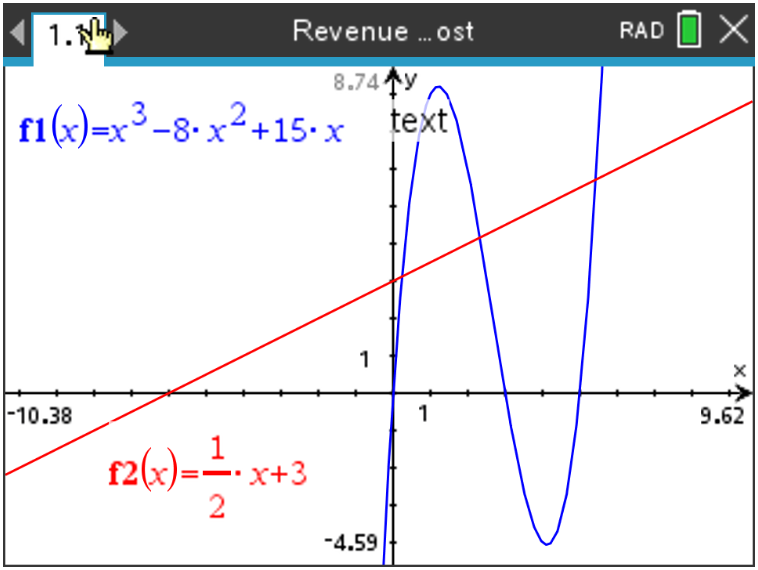# Activities

•• ##### Subject Area

• Math: Calculus: Derivatives
• Math: Calculus: Definite Integrals and Applications
• Math: Calculus: Applications of the Derivative

9-12

60 Minutes

• ##### Device
•TI-Nspire™ CX/CX II
•TI-Nspire™ CX CAS/CX II CAS
• ##### Software

TI-Nspire™ CX CAS
TI-Nspire™ CX

5.2

## Profit Equations and Calculus#### Activity Overview

In this activity, students will discuss the graphical and algebraic relationships between a function and its first and second derivatives, namely a profit function.

#### Objectives

• Students will further discuss the graphical and algebraic relationships between a function and its first and second derivatives, namely a profit function.
• Students will use their knowledge of Optimization to maximize profits or minimize losses.
• Students will use Integration to find a profit function given its rate of change.
•  Students will try to make a connection with how to understand these topics in IB Mathematics courses and on their final assessments.

#### Vocabulary

• Cost
• Revenue
• Optimization
• Profit
• Differentiation
• Integration